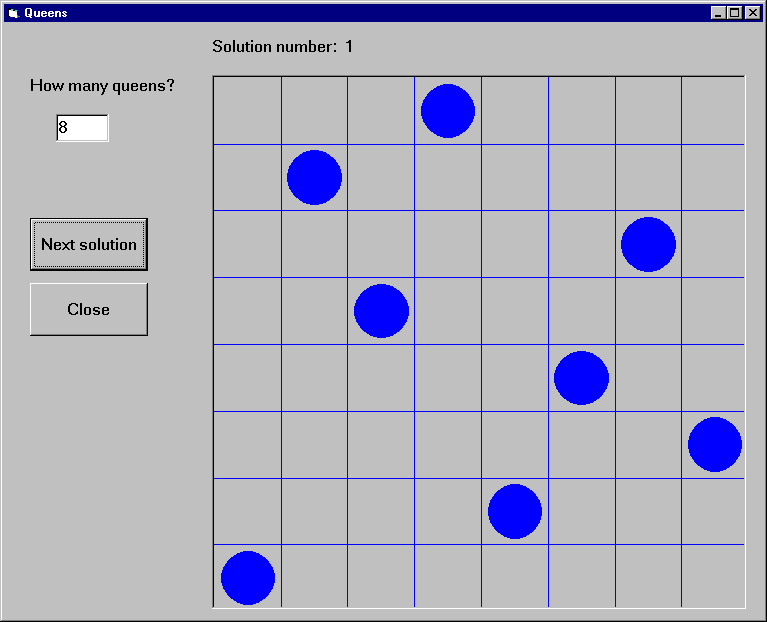#### 46.4.3 Example 3 - Queens

This example gives a Visual Basic user interface to an N-queens program. The purpose of this example is to show how to handle Prolog lists through the Visual Basic interface. The full source code of the example is found in the distribution.

The user interface shown in this example will allow the user to specify the number of queens, and, with the help of the `Next Solution` command button all the solutions of the N-Queens problem will be enumerated. A given solution will be represented in a simple graphical way as a PictureBox, using the basic `Circle` and `Line` methods.The problem itself will be solved in Prolog, using a `queens(`+N`,`?PositionList`)` Prolog predicate, stored in the file queens.

We now present two solutions, using different techniques for retrieving Prolog lists.

Example 3a - N-Queens, generating a variable list into the Prolog call

The first implementation of the N-Queens problem is based on the technique of generating a given length list of Prolog variables into the Prolog query.

For example, if the N-Queens problem is to be solved for N = 4, i.e. with the query "`queens(4,L)`", then the problem of retrieving a list from Visual Basic will arise. However, if the query is presented as "`queens(4,[X1,X2,X3,X4])`", then instead of retrieving the list it is enough to access the `X1,X2,X3,X4` values. Since the number of queens is not fixed in the program, this query has to be generated, and the retrieval of the Xi values must be done in a cycle.

This approach can always be applied when the format of the solution is known at the time of calling the query.

We now go over the complete code of the program.

Global declarations used in the program (General/declarations):

```     Dim nQueens As Long       'number of queens
Dim nSol As Long          'index of solution
Dim nActqid As Long       'actual query identifier
Dim nQueryOpen As Boolean 'there is an open query
```

The initialization of the program will be done when the form window is loaded:

```     Private Sub Form_Load()
nQueens = 0
nSol = 1
nQueryOpen = False

'initialize Prolog
If PrologInit() <> 1 Then GoTo Err
If PrologQueryCutFail("load_files(app(queens))") <> 1 Then GoTo Err
Exit Sub

Err:
End Sub
```

Deinitialization of the Prolog engine will be done when the form windows is closed, exactly as for the calculator example.

When the number of queens changes (i.e. the value of the text box `textSpecNo` changes), a new query has to be opened, after the previous query, if there has been any, is closed.

```     Private Sub textSpecNo_Change()
nQueens = Val(textSpecNo)
nSol = 1

If nQueryOpen Then PrologCloseQuery (nActqid)

'create Prolog query in form: queens(4,[X1,X2,X3,X4])

Q = "queens(" & Str(nQueens) & ", ["
For i = 1 To nQueens - 1 Step 1
Q = Q & "X" & i & ","
Next
Q = Q & "X" & nQueens & "])"

nActqid = PrologOpenQuery(Q)
nQueryOpen = True
End Sub
```

The `Next command` button executes and shows the next solution of the current query:

```     Private Sub cmdNext_Click()
Dim nPos As Long
Dim aPos(100) As Long

If Not nQueryOpen Then
MsgBox "Specify number of queens first!", 48, ""
Exit Sub
End If
If PrologNextSolution(nActqid) < 1 Then
MsgBox "No more solutions!", 48, ""
Else
For i = 1 To nQueens Step 1
If PrologGetLong(nActqid, "X" & i, nPos) = 1 Then
aPos(i - 1) = nPos
End If
Next i

'display nth solution
txtSolNo = "Solution number: " & Str(nSol)
Call draw_grid(nQueens)

nLine = 1
For Each xElem In aPos
Call draw_circle(nLine, xElem, nQueens)
nLine = nLine + 1
Next

nSol = nSol + 1
End If
End Sub
```

Drawing itself is performed by the `draw_grid` and `draw_circle` procedures.

Example 3b - N-Queens, converting the resulting Prolog list to an atom

The second variant of the N-Queens program uses the technique of converting the resulting Prolog list into a string via the `PrologGetString` function, and decomposing it into an array in Visual Basic. Here we show only those parts of the program that have changed with respect to the first version.

In the `textSpecNo_Change` routine the `queens/2` predicate is called with a single variable in its second argument:

```     Q = "queens(" & Str(nQueens) & ",Queens)"
nActqid = PrologOpenQuery(Q)
```

In the `cmdNext_Click` routine the solution list is retrieved into a single string, which is then split up along the commas, and deposited into the `aPos` array by the `convert_prolog_list` routine. (`aPos` is now an array of strings, rather than integers.)

Finally, we include the code of the routine for splitting up a Prolog list:

```     Private Sub convert_prolog_list(ByVal inList As String,
ByRef inArray() As String)
'drop brackets
xList = Mid(inList, 2, Len(inList) - 2)

i = 0

startPos = 1
xList = Mid(xList, startPos)

Do While xList <> ""
endPos = InStr(xList, ",")
If endPos = 0 Then
xElem = xList
inArray(i) = xElem
Exit Do
End If
xElem = Mid(xList, 1, endPos - 1)
inArray(i) = xElem
i = i + 1
xList = Mid(xList, endPos + 1)
startPos = endPos + 1
Loop
End Sub
```